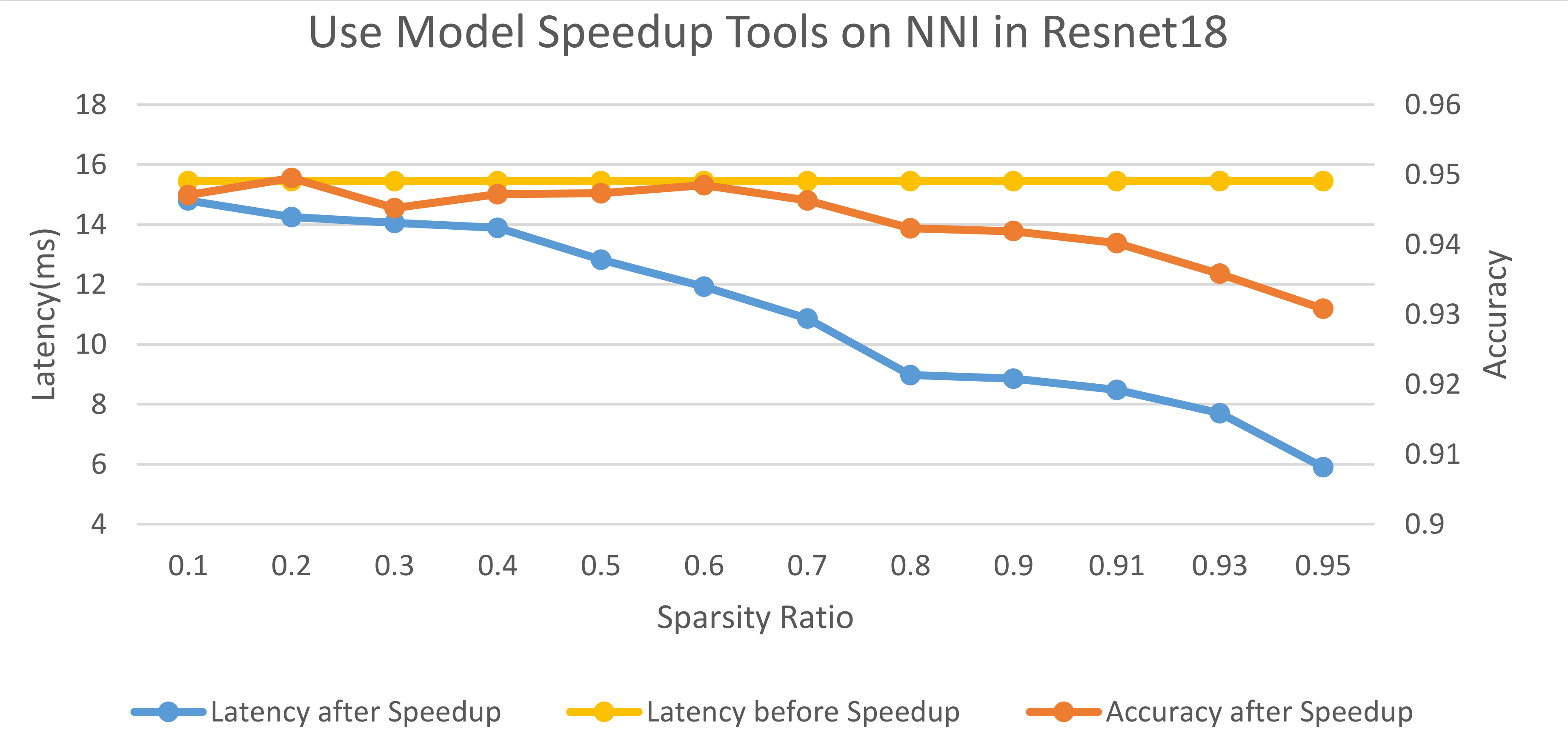This feature is in Beta version.

## Introduction¶

Pruning algorithms usually use weight masks to simulate the real pruning. Masks can be used to check model performance of a specific pruning (or sparsity), but there is no real speedup. Since model speedup is the ultimate goal of model pruning, we try to provide a tool to users to convert a model to a smaller one based on user provided masks (the masks come from the pruning algorithms).

There are two types of pruning. One is fine-grained pruning, it does not change the shape of weights, and input/output tensors. Sparse kernel is required to speed up a fine-grained pruned layer. The other is coarse-grained pruning (e.g., channels), shape of weights and input/output tensors usually change due to such pruning. To speed up this kind of pruning, there is no need to use sparse kernel, just replace the pruned layer with smaller one. Since the support of sparse kernels in community is limited, we only support the speedup of coarse-grained pruning and leave the support of fine-grained pruning in future.

## Design and Implementation¶

To speed up a model, the pruned layers should be replaced, either replaced with smaller layer for coarse-grained mask, or replaced with sparse kernel for fine-grained mask. Coarse-grained mask usually changes the shape of weights or input/output tensors, thus, we should do shape inference to check are there other unpruned layers should be replaced as well due to shape change. Therefore, in our design, there are two main steps: first, do shape inference to find out all the modules that should be replaced; second, replace the modules. The first step requires topology (i.e., connections) of the model, we use `jit.trace` to obtain the model graph for PyTorch.

For each module, we should prepare four functions, three for shape inference and one for module replacement. The three shape inference functions are: given weight shape infer input/output shape, given input shape infer weight/output shape, given output shape infer weight/input shape. The module replacement function returns a newly created module which is smaller.

## Usage¶

```from nni.compression.pytorch import ModelSpeedup
# model: the model you want to speed up
# dummy_input: dummy input of the model, given to `jit.trace`
m_speedup.speedup_model()
dummy_input = dummy_input.to(device)
start = time.time()
out = model(dummy_input)
print('elapsed time: ', time.time() - start)
```

For complete examples please refer to the code

NOTE: The current implementation supports PyTorch 1.3.1 or newer.

## Limitations¶

Since every module requires four functions for shape inference and module replacement, this is a large amount of work, we only implemented the ones that are required by the examples. If you want to speed up your own model which cannot supported by the current implementation, you are welcome to contribute.

For PyTorch we can only replace modules, if functions in `forward` should be replaced, our current implementation does not work. One workaround is make the function a PyTorch module.

## Speedup Results of Examples¶

The code of these experiments can be found here.

### slim pruner example¶

on one V100 GPU, input tensor: `torch.randn(64, 3, 32, 32)`

Times

Speedup Latency

1

0.01197

0.005107

2

0.02019

0.008769

4

0.02733

0.014809

8

0.04310

0.027441

16

0.07731

0.05008

32

0.14464

0.10027

### fpgm pruner example¶

on cpu, input tensor: `torch.randn(64, 1, 28, 28)`, too large variance

Times

Speedup Latency

1

0.01383

0.01839

2

0.01167

0.003558

4

0.01636

0.01088

40

0.14412

0.08268

40

1.29385

0.14408

40

0.41035

0.46162

400

6.29020

5.82143

### l1filter pruner example¶

on one V100 GPU, input tensor: `torch.randn(64, 3, 32, 32)`

Times

Speedup Latency

1

0.01026

0.003677

2

0.01657

0.008161

4

0.02458

0.020018

8

0.03498

0.025504

16

0.06757

0.047523

32

0.10487

0.086442

### APoZ pruner example¶

on one V100 GPU, input tensor: `torch.randn(64, 3, 32, 32)`

Times

Speedup Latency

1

0.01389

0.004208

2

0.01628

0.008310

4

0.02521

0.014008

8

0.03386

0.023923

16

0.06042

0.046183

32

0.12421

0.087113

### SimulatedAnnealing pruner example¶

In this experiment, we use SimulatedAnnealing pruner to prune the resnet18 on the cifar10 dataset. We measure the latencies and accuracies of the pruned model under different sparsity ratios, as shown in the following figure. The latency is measured on one V100 GPU and the input tensor is `torch.randn(128, 3, 32, 32)`.### User configuration for ModelSpeedup¶

PyTorch

class nni.compression.pytorch.ModelSpeedup(model, dummy_input, masks_file, map_location=None, batch_dim=0, confidence=8)[source]

This class is to speedup the model with provided weight mask.

Parameters
• model (pytorch model) – The model user wants to speed up

• dummy_input (pytorch tensor, tuple of tensor, list of tensor) – Note: The first dimension of the dummy_input should be the batchsize. The dummy input for ``jit.trace``, users should put it on the right device.

• map_location (str) – the device on which masks are placed, same to map_location in ``torch.load``